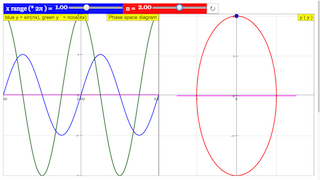Intro Page

# Phase space diagram

Phase space characterizes the interdependence of the variables and their derivatives in a given function y = y(x), for example y = y (x, y´,y´´). 2 dimensional phase space diagrams are projections of phase space onto a plane, as y = y (y´) or y´= y´(y´´). In 3D visualizations the projection can be spatial as in y = y( y´,y´´).

Phase space projections often characterize a function very evidently. In this simple simulation this is demonstrated for the periodicity of the sine function.

The left window shows the function y = sin (n x) itself in blue, its first derivative y´= ncos (nx) in green. The zero line is in magenta. With the slider n you can change the parameter n, which defines the number of periods in an x interval of 2π. Reset defines n = 1.

A second slider x range defines the range of x in the left window between 0.1 and 5 times . Default value is 0.5, limiting the range of the function to one half period.

The right window shows the phase projection y´(y) in red. A blue point characterizes the relation y´(y) at the end of the x range. At default (n = 1 and xrange/2π=0.5) the phase space diagram is a half circle, corresponding to a half period of the function within the xrange. When xrange/2π is decreased, the phase space curve will contract. xrange/2π=1.0 leads to a full circle.

With n ≠ 1 the derivative has an amplitude n times that of the function. Correspondingly the phase diagram becomes an ellipse with an elongation of n. It has as many revolutions as the function has periods in the interval.

Periodicity is characterized by a closed space phase diagram with as many cycles as the function has periods.

### Translations

Code Language Translator Run### Software Requirements

SoftwareRequirements

 Android iOS Windows MacOS with best with Chrome Chrome Chrome Chrome support full-screen? Yes. Chrome/Opera No. Firefox/ Samsung Internet Not yet Yes Yes cannot work on some mobile browser that don't understand JavaScript such as..... cannot work on Internet Explorer 9 and below

### CreditsDieter Roess - WEH- Foundation; Fremont Teng; Loo Kang Wee

[text]

## Phase Space Diagram JavaScript Simulation Applet HTML5

### Instructions

#### x-range and n Sliders

Adjusting the blue slider will change the x range,
while the red slider will affect the position n of the object in the right diagram.

#### Toggling Full Screen

Double clicking anywhere in the panels will toggle full screen.

#### Reset Button

Resets the simulation.

Research

[text]

[text]

[text]

### end faq